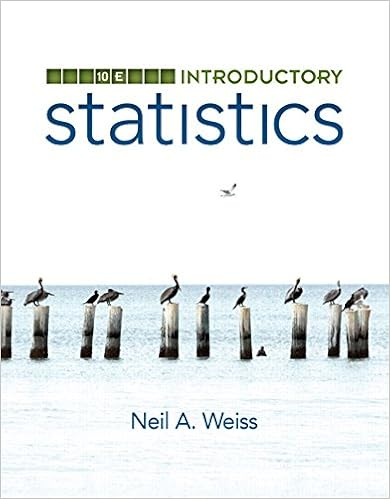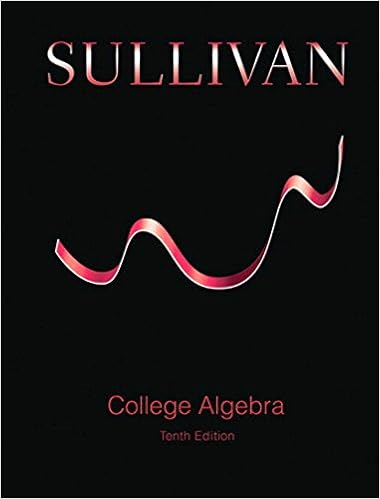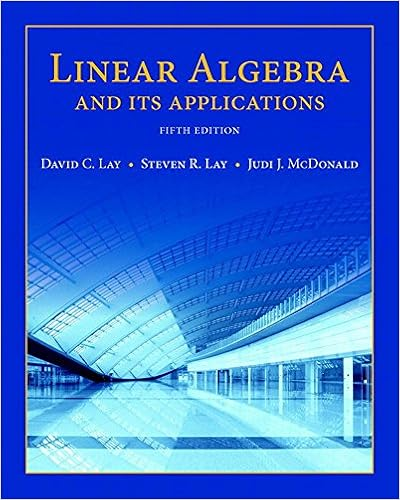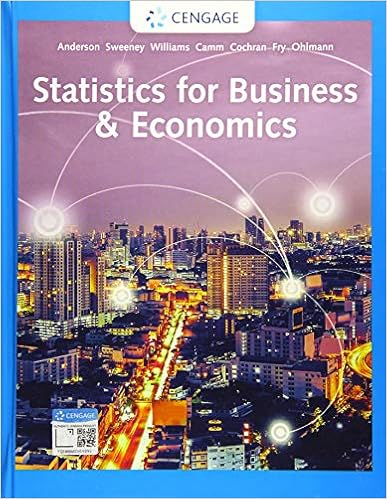# Midterm 2 Exam - Midterm II Laurence Field Math 152,...

• CountAtomAardvark3912
• 7
• 100% (1) 1 out of 1 people found this document helpful

This preview shows page 1 - 4 out of 7 pages.

The preview shows page 2 - 4 out of 7 pages.
Midterm IILaurence FieldMath 152, Section 31February 24, 2012Name:Instructions:This midterm has 6 questions for a total of 60 points.The value of each part of each question is stated.No books, notes or calculators are allowed.Please show your working.Partial credit will be awarded to partially correct answers so long as your working is legible.QuestionPointsScore110210310410510610Total:60i
##### We have textbook solutions for you!The document you are viewing contains questions related to this textbook.
Chapter 6 / Exercise 1
Calculus
StewartExpert VerifiedBrowse all Textbook Solutions
1. Compute the following values.(a) (2 points)Ze511tdt(b) (4 points) The average value of the functionf(x) =1x2on the interval [a, b], where 0< a < b.3
(c) (4 points)-πxcosx+ 1dx1
2.(a) (3 points) What does it mean to say “the functionfis one-to-one”?(b) (3 points) Give the definition and the derivative of the natural logarithm function,L(x) = log(x) =loge(x) = ln(x) (using whichever notation you prefer).(c) (2 points) Iffis a continuous function on [a, b], which of the following is necessarily true?Circle youranswer. No justification needed.(i)Zbaf(x)dxZba|f(x)|dx(ii)Zbaf(x)dx=Zba|f(x)|dx(iii)Zbaf(x)dxZba|

Course Hero member to access this document

Course Hero member to access this document

End of preview. Want to read all 7 pages?

Course Hero member to access this document

Term
Fall
Professor
townsend
Tags
Math, Continuous function, Inverse function, Logarithm
##### We have textbook solutions for you!
The document you are viewing contains questions related to this textbook.The document you are viewing contains questions related to this textbook.
Chapter 6 / Exercise 1
Calculus
StewartExpert Verified
•••